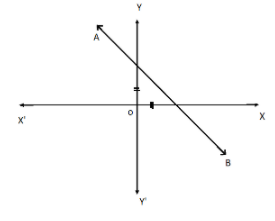QuestionAnswers

# In each of the following, find the inclination of line AB:Verified
91.5k+ views
Hint: Here, we will first find the value of the sum of the angles of the formed triangle. Then by using the concept of the congruent sides we will find the value of one of the angles of the triangle with which the line AB is inclined.

From the figure line AB is passing through both the axis X-axis and Y-axis. The line AB forms a triangle with the $x$- axis and the $y$– axis as it intersects both the axis at two points.
We can clearly see that in the formed triangle, the angle between the X-axis and the Y-axis is $90^\circ$.
We know that the sum of all the interior angles of a triangle is $180^\circ$.
Hence the sum of the remaining two angles of the triangle will be equal to $90^\circ$.
From the figure, we can see that two sides of the triangle formed are congruent i.e. those sides are equal. Therefore, by the concept of the congruent, the opposite angles to these sides will be equal and the sum of those angles is equal to $90^\circ$.
Hence the value of both the angles will be $45^\circ$.
We know that the inclination of a line AB is the angle it makes with the $x$- axis which is equal to $45^\circ$.
Hence, inclination of line AB is $45^\circ$.
Note: We should remember that the congruent sides means whenever the two sides of a triangle are equal then the angles opposite to the sides of the triangle are also equal. In a Cartesian plane, the angle between the X-axis and the Y-axis is always equal to $90^\circ$ i.e. both the axes are at the right angle to each other. The X-axis is the horizontal axis in the Cartesian plane and Y-axis is the vertical axis in the Cartesian plane. The inclination of a line or object is always taken in reference to the X-axis or horizontal plane.